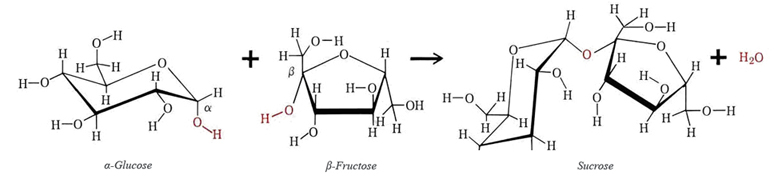# 2.5.3.2: Disaccharides

$$\newcommand{\vecs}{\overset { \rightharpoonup} {\mathbf{#1}} }$$ $$\newcommand{\vecd}{\overset{-\!-\!\rightharpoonup}{\vphantom{a}\smash {#1}}}$$$$\newcommand{\id}{\mathrm{id}}$$ $$\newcommand{\Span}{\mathrm{span}}$$ $$\newcommand{\kernel}{\mathrm{null}\,}$$ $$\newcommand{\range}{\mathrm{range}\,}$$ $$\newcommand{\RealPart}{\mathrm{Re}}$$ $$\newcommand{\ImaginaryPart}{\mathrm{Im}}$$ $$\newcommand{\Argument}{\mathrm{Arg}}$$ $$\newcommand{\norm}{\| #1 \|}$$ $$\newcommand{\inner}{\langle #1, #2 \rangle}$$ $$\newcommand{\Span}{\mathrm{span}}$$ $$\newcommand{\id}{\mathrm{id}}$$ $$\newcommand{\Span}{\mathrm{span}}$$ $$\newcommand{\kernel}{\mathrm{null}\,}$$ $$\newcommand{\range}{\mathrm{range}\,}$$ $$\newcommand{\RealPart}{\mathrm{Re}}$$ $$\newcommand{\ImaginaryPart}{\mathrm{Im}}$$ $$\newcommand{\Argument}{\mathrm{Arg}}$$ $$\newcommand{\norm}{\| #1 \|}$$ $$\newcommand{\inner}{\langle #1, #2 \rangle}$$ $$\newcommand{\Span}{\mathrm{span}}$$

The sugar molecules listed in Figure $$\PageIndex{1}$$ are usually referred to as monosaccharides. This distinguishes them from the disaccharides which are made up by condensing two sugar units.Figure $$\PageIndex{1}$$ The linear form of some important monosaccharides: (a) ribose; (b) fructose; (c) mannose; (d) glucose.

A familiar example of a disaccharide is ordinary cane sugar, sucrose, which may be obtained by condensing a molecule of α-glucose with one of the cyclic forms of fructose called β-fructose. The structure of sucrose is shown in Figure $$\PageIndex{2}$$.Figure $$\PageIndex{2}$$ The formation of sucrose from glucose and fructose.

Other, less familiar, examples of disaccharides are lactose, which occurs in milk, and maltose, which are shown in Figure$$\PageIndex{3}$$. In order to digest a disaccharide like sucrose or lactose, the human body must have an enzyme which can catalyze hydrolysis of the linkage between the two monosaccharide units. Many Asians, Africans, and American Indians are incapable of synthesizing lactase, the enzyme that speeds hydrolysis of lactose. If such persons drink milk, the undigested lactose makes them sick.Figure $$\PageIndex{3}$$ Two other common disaccharides, maltose and lactose.